# Python | Pandas Index.identical()

Python is a great language for doing data analysis, primarily because of the fantastic ecosystem of data-centric python packages. Pandas is one of those packages and makes importing and analyzing data much easier.

Pandas` Index.identical()` function determine if two Index objects contains the same elements. If they contain the same elements then the function returns `True` else the function returns `False` indicating the values contained in both the Indexes are different. This is similar to the `Index.equals()`, but check that other comparable attributes are also equal.

Syntax: Index.identical(other)

Parameters :
Other : index

Returns : boolean value

Example #1: Use `Index.identical()` function to check if two Indexes contain same elements and if other attributes are identical or not.

 `# importing pandas as pd ` `import` `pandas as pd ` ` `  `# Creating the first Index ` `idx1 ``=` `pd.Index([``'Labrador'``, ``'Beagle'``, ``'Labrador'``, ``'Lhasa'``, ``'Husky'``, ``'Beagle'``]) ` ` `  `# Creating the second Index ` `idx2 ``=` `pd.Index([``'Labrador'``, ``'Beagle'``, ``'Pug'``, ``'Lhasa'``, ``'Husky'``, ``'Pitbull'``]) ` ` `  `# Print the first and second Index ` `print``(idx1, ``'\n'``, idx2) `

Output :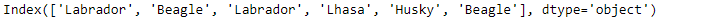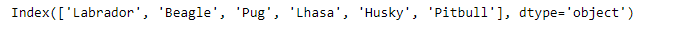Let’s check if the two Indexes are identical or not.

 `# Checking the equality of the two Indexes ` `idx1.identical(idx2) `

Output :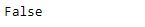As we can see in the output, the `Index.identical()` function has returned `False` indicating that the Indexes are not equal.

Example #2: Use `Index.identical()` function to check if the input indexes are identical to each other or not.

 `# importing pandas as pd ` `import` `pandas as pd ` ` `  `# Creating the first Index ` `idx1 ``=` `pd.Index([``'Jan'``, ``'Feb'``, ``'Mar'``, ``'Apr'``, ``'May'``, ``'Jun'``, ` `                ``'Jul'``, ``'Aug'``, ``'Sep'``, ``'Oct'``, ``'Nov'``, ``'Dec'``]) ` ` `  `# Creating the second Index ` `idx2 ``=` `pd.Index([``'Jan'``, ``'Feb'``, ``'Mar'``, ``'Apr'``, ``'May'``, ``'Jun'``, ` `                ``'Jul'``, ``'Aug'``, ``'Sep'``, ``'Oct'``, ``'Nov'``, ``'Dec'``]) ` ` `  `# Print the first and second Index ` `print``(idx1, ``'\n'``, idx2) `

Output :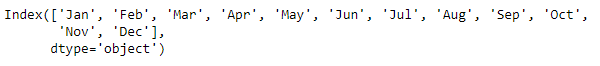Let’s check if the two Indices are identical to each other or not.

 `# test the equality ` `idx1.identical(idx2) `

Output :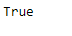The function has returned `True` indicating that both the Indexes are identical to each other.

My Personal Notes arrow_drop_upCheck out this Author's contributed articles.

If you like GeeksforGeeks and would like to contribute, you can also write an article using contribute.geeksforgeeks.org or mail your article to contribute@geeksforgeeks.org. See your article appearing on the GeeksforGeeks main page and help other Geeks.

Please Improve this article if you find anything incorrect by clicking on the "Improve Article" button below.

Article Tags :

Be the First to upvote.

Please write to us at contribute@geeksforgeeks.org to report any issue with the above content.